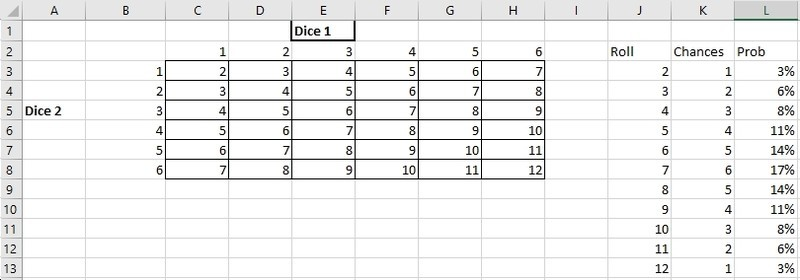# Don’t Take A Shot in the Dark: Using Excel to Calculate Probabilities

In today’s world, everyone is focused on the latest technological innovation and how it will change our lives. However, when it comes to the value of technology, not everything has to be as complex or immersive as blockchain or virtual reality. For Windows users, a simple Excel spreadsheet can be a powerful tool if used correctly. Even though it won’t change the world or make the headlines, Excel has been proving its worth in the computing world since 1985 (1987 for Windows systems). Beyond creating invoices and tables, Excel is perfect for completing calculations.

By lining up dozens of variables and scenarios, Excel can tell you the exact probabilities of an event and, moreover, adjust them in real time as certain values change. To show you what we mean, here’s an overview of how you can use Microsoft Excel to calculate probabilities.

## Looking at Dice Rolls in Excel

Load up Excel (Start>Programs>Microsoft Office>Microsoft Excel) and insert two headings in two separate columns. These headings should relate to the variables you want to assess. For example, if you wanted to know the probability of rolling a certain number with two dice, you’d first create a mini table in the following way:

“Dice 1” – Vertical Axis (from cell C2 to H2): In these cells, you would put all the possible outcomes from a single dice roll i.e. 1 through to 6.

“Dice 2” – Horizontal Axis (from cell C2 to C7): in these cells, you would put all the possible outcomes from a single dice roll i.e. 1 through to 6.

Once you’ve done that, start listing all the possible totals you can make when you combine the vertical and horizontal axis. See image below.

After this, you can input the following data under separate headings:

Roll: Start in cell J3. This relates the totals you can hit with two die and ranges from 2 to 12.

Chances: Start in K3. This relates to the chances of hitting each number and takes into account the range of variables in play. Then, in this column, you can input the formula =COUNTIF(\$C\$3:\$H\$8,J3). In other words, this formula tells you the number of ways it’s possible to roll a certain total given the contents of your table.

*Don’t worry if you get a #NUM! error because Microsoft can help you correct the mistake.

Probability: Start in cell H3. In this column, you can input the following formula and it will add up all the chances and divide by the number of outcomes that can happen. In this example that are 36 possible outcomes. The figure shown in this box is the probability of rolling a certain number.

## Analyse Everything from Lotteries to Raffles and More

So, why is this useful? Well, it’s an easy way to work out the probability of certain popular events. For example, if you wanted to know the probability of winning Powerball draws, you can take the variables listed and apply the same technique. For instance, according to the rules of the game, there are 69 numbers and 26 Powerballs in play. To win the jackpot, you need to match five numbers and the Powerball.

If you create the table shown below and input this formula “=COMBIN(A3,C3)*COMBIN(B3,D3)” into the “probability” column, you find that the chances of winning the Powerball jackpot are 1 in 292 million.Taking this a step further, you could look at the probability of winning a raffle. Let’s say there are 100 possible numbers/tickets and you’ve bought five. In this scenario, your chances of winning are 0.1 or 10%. To get this, create the table below and simply use the formula =A2/B2 in the probability column. Once you’ve done that, highlight the probabilities, click the % button and Excel will convert everything for you.So, there you have it. In simple terms, the probability of something happening is the number of chances divided by the total number of possible outcomes. As long as you know the range of possible outcomes and the outcomes you want to focus on, you can plug the data into Excel. Indeed, if you’ve ever wondered what the chances were of a certain event happening, you now know you can use a simple spreadsheet to get the answer instead of taking a shot in the dark.